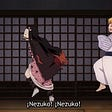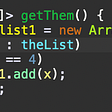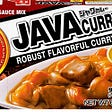# 0. What is DMII?

`Shader "DMIIShader" {    Properties {        _MainTex("Texture", 2D) = "white" {}    }    SubShader {        Tags {            "Queue" = "Transparent"        }        Cull Off        Lighting Off        ZWrite Off        Blend SrcAlpha OneMinusSrcAlpha​        Pass {            CGPROGRAM            #pragma vertex vert            #pragma fragment frag            #pragma multi_compile_instancing            #include "UnityCG.cginc"            #pragma instancing_options procedural:setup​            struct vertex {                float4 loc  : POSITION;                float2 uv   : TEXCOORD0;                UNITY_VERTEX_INPUT_INSTANCE_ID            };            struct fragment {                float4 loc  : SV_POSITION;                float2 uv   : TEXCOORD0;            };                        CBUFFER_START(MyData)                float4 posDirBuffer;            CBUFFER_END                #ifdef UNITY_PROCEDURAL_INSTANCING_ENABLED            void setup() {                float2 position = posDirBuffer[unity_InstanceID].xy;                float2 direction = posDirBuffer[unity_InstanceID].zw;​                unity_ObjectToWorld = float4x4(                    direction.x, -direction.y, 0, position.x,                    direction.y, direction.x, 0, position.y,                    0, 0, 1, 0,                    0, 0, 0, 1                    );            }    #endif​            sampler2D _MainTex;            float _FadeInT; //We'll use this later​            fragment vert(vertex v) {                fragment f;                UNITY_SETUP_INSTANCE_ID(v);                f.loc = UnityObjectToClipPos(v.loc);                f.uv = v.uv;                //f.uv = TRANSFORM_TEX(v.uv, _MainTex);                return f;            }​            float4 frag(fragment f) : SV_Target{                float4 c = tex2D(_MainTex, f.uv);                return c;            }            ENDCG        }    }}`
`#pragma vertex vert#pragma fragment frag#pragma multi_compile_instancing#include "UnityCG.cginc"#pragma instancing_options procedural:setup`
`struct vertex {    float4 loc  : POSITION;    float2 uv   : TEXCOORD0;    UNITY_VERTEX_INPUT_INSTANCE_ID};`
`CBUFFER_START(MyData)    float4 posDirBuffer;    float timeBuffer;CBUFFER_END`
`#ifdef UNITY_PROCEDURAL_INSTANCING_ENABLED        void setup() {            float2 position = posDirBuffer[unity_InstanceID].xy;            float2 direction = posDirBuffer[unity_InstanceID].zw;​            unity_ObjectToWorld = float4x4(                direction.x, -direction.y, 0, position.x,                direction.y, direction.x, 0, position.y,                0, 0, 1, 0,                0, 0, 0, 1                );        }#endif`
`fragment vert(vertex v) {    UNITY_SETUP_INSTANCE_ID(v);    fragment f;    f.loc = UnityObjectToClipPos(v.loc);    f.uv = v.uv;    //f.uv = TRANSFORM_TEX(v.uv, _MainTex);    f.c = float4(1.0, 1.0, 1.0, 1.0);    return f;}`

# 2. Mesh and Material

`public readonly struct RenderInfo {    private static readonly int MainTexPropertyId = Shader.PropertyToID("_MainTex");    public readonly Mesh mesh;    public readonly Material mat;​    public RenderInfo(Mesh m, Material material) {        mesh = m;        mat = material;    }​    public static RenderInfo FromSprite(Material baseMaterial, Sprite s) {        var renderMaterial = UnityEngine.Object.Instantiate(baseMaterial);        renderMaterial.enableInstancing = true;        renderMaterial.SetTexture(MainTexPropertyId, s.texture);        Mesh m = new Mesh {            vertices = s.vertices.Select(v => (Vector3)v).ToArray(),            triangles = s.triangles.Select(t => (int)t).ToArray(),            uv = s.uv        };        return new RenderInfo(m, renderMaterial);    }}`

# 3.1 An Object

`public class FObject {        private static readonly Random r = new Random();        public Vector2 position;        public readonly float scale;        private readonly Vector2 velocity;        public float rotation;        private readonly float rotationRate;        public float time;​        public FObject() {            position = new Vector2((float)r.NextDouble() * 10f - 5f, (float)r.NextDouble() * 8f - 4f);            velocity = new Vector2((float)r.NextDouble() * 0.4f - 0.2f, (float)r.NextDouble() * 0.4f - 0.2f);             rotation = (float)r.NextDouble();            rotationRate = (float)r.NextDouble() * 0.6f - 0.2f;            scale = 0.6f + (float) r.NextDouble() * 0.8f;            time = (float) r.NextDouble() * 6f;        }​        public void DoUpdate(float dT) {            position += velocity * dT;            rotation += rotationRate * dT;            time += dT;        }    }`

# 3.2 A Manager

`private static readonly int posDirPropertyId = Shader.PropertyToID("posDirBuffer");    private static readonly int timePropertyId = Shader.PropertyToID("timeBuffer");        private MaterialPropertyBlock pb;    private readonly Vector4[] posDirArr = new Vector4[batchSize];    private readonly float[] timeArr = new float[batchSize];    private const int batchSize = 7;    public int instanceCount;        public Sprite sprite;    public Material baseMaterial;    private RenderInfo ri;    public string layerRenderName;    private int layerRender;    private FObject[] objects;...`
`private void Start() {    pb = new MaterialPropertyBlock();    layerRender = LayerMask.NameToLayer(layerRenderName);    ri = RenderInfo.FromSprite(baseMaterial, sprite);    Camera.onPreCull += RenderMe;    objects = new FObject[instanceCount];    for (int ii = 0; ii < instanceCount; ++ii) {        objects[ii] = new FObject();    }}​private void Update() {    float dT = Time.deltaTime;    for (int ii = 0; ii < instanceCount; ++ii) {        objects[ii].DoUpdate(dT);    }}`
`private void RenderMe(Camera c) {    if (!Application.isPlaying) { return; }    for (int done = 0; done < instanceCount; done += batchSize) {        int run = Math.Min(instanceCount - done, batchSize);        for (int batchInd = 0; batchInd < run; ++batchInd) {            var obj = objects[done + batchInd];            posDirArr[batchInd] = new Vector4(obj.position.x, obj.position.y,                 Mathf.Cos(obj.rotation) * obj.scale, Mathf.Sin(obj.rotation) * obj.scale);            timeArr[batchInd] = obj.time;        }        pb.SetVectorArray(posDirPropertyId, posDirArr);        pb.SetFloatArray(timePropertyId, timeArr);        CallRender(c, run);    }}`
`private void CallRender(Camera c, int count) {    Graphics.DrawMeshInstancedProcedural(ri.mesh, 0, ri.mat,        bounds: new Bounds(Vector3.zero, Vector3.one * 1000f),        count: count,        properties: pb,        castShadows: ShadowCastingMode.Off,        receiveShadows: false,        layer: layerRender,        camera: c);}`

`Properties {    _MainTex("Texture", 2D) = "white" {}    _FadeInT("Fade in time", Float) = 10    // New}`
`CBUFFER_START(MyData)    float4 posDirBuffer;    float timeBuffer; // NewCBUFFER_END`
`struct fragment {    float4 loc  : SV_POSITION;    float2 uv   : TEXCOORD0;    UNITY_VERTEX_INPUT_INSTANCE_ID          // New};fragment vert(vertex v) {    fragment f;    UNITY_SETUP_INSTANCE_ID(v);    UNITY_TRANSFER_INSTANCE_ID(v, f);       // New    f.loc = UnityObjectToClipPos(v.loc);    f.uv = v.uv;    //f.uv = TRANSFORM_TEX(v.uv, _MainTex);    return f;}float4 frag(fragment f) : SV_Target{    UNITY_SETUP_INSTANCE_ID(f);             // New    float4 c = tex2D(_MainTex, f.uv);    return c;}`
`float _FadeInT;                                                         // New​float4 frag(fragment f) : SV_Target{    UNITY_SETUP_INSTANCE_ID(f);    float4 c = tex2D(_MainTex, f.uv);#if defined(UNITY_PROCEDURAL_INSTANCING_ENABLED) || defined(UNITY_INSTANCING_ENABLED)                           // New    c.a *= smoothstep(0.0, _FadeInT, timeBuffer[unity_InstanceID]);     // New#endif                                                                  // New    return c;}`

# 5. But What If My Computer Is From 2005?

• For DrawMeshIndirectInstanced/Procedural, the position matrix for each element is created in the `setup()` function in the shader, which is run on the GPU. Calling either of these functions sets `UNITY_PROCEDURAL_INSTANCING_ENABLED`.
• For DrawMeshInstanced, the position matrix for each element must be computed in C# (on the CPU) and provided to the function call as an array. Calling this function sets `UNITY_INSTANCING_ENABLED`.
• You may be wondering why we need to check for the flags at all if the shader isn’t designed to work with non-instancing use cases. The problem is that references to constructs like `unity_InstanceID` will cause compilation errors if not enclosed within a flag check, and this may or may not cause compilation issues with your project at large.
`private readonly Vector4[] posDirArr = new Vector4[batchSize];private readonly float[] timeArr = new float[batchSize];private readonly Matrix4x4[] posMatrixArr = new Matrix4x4[batchSize]; //New`
`private void RenderMe(Camera c) {    if (!Application.isPlaying) { return; }    for (int done = 0; done < instanceCount; done += batchSize) {        int run = Math.Min(instanceCount - done, batchSize);        for (int batchInd = 0; batchInd < run; ++batchInd) {            var obj = objects[done + batchInd];            //posDirArr[batchInd] = new Vector4(obj.position.x, obj.position.y,             //    Mathf.Cos(obj.rotation) * obj.scale, Mathf.Sin(obj.rotation) * obj.scale);            timeArr[batchInd] = obj.time;            ref var m = ref posMatrixArr[batchInd];​            m.m00 = m.m11 = Mathf.Cos(obj.rotation) * obj.scale;            m.m01 = -(m.m10 = Mathf.Sin(obj.rotation) * obj.scale);            m.m22 = m.m33 = 1;            m.m03 = obj.position.x;            m.m13 = obj.position.y;        }        //pb.SetVectorArray(posDirPropertyId, posDirArr);        pb.SetFloatArray(timePropertyId, timeArr);        //CallRender(c, run);        CallLegacyRender(c, run);    }}`
`//Use this for legacy GPU support or WebGL supportprivate void CallLegacyRender(Camera c, int count) {    Graphics.DrawMeshInstanced(ri.mesh, 0, ri.mat,        posMatrixArr,        count: count,        properties: pb,        castShadows: ShadowCastingMode.Off,        receiveShadows: false,        layer: layerRender,        camera: c);}`
`#ifdef UNITY_PROCEDURAL_INSTANCING_ENABLED        void setup() {            float2 position = posDirBuffer[unity_InstanceID].xy;            float2 direction = posDirBuffer[unity_InstanceID].zw;            direction *= smoothstep(0, 10, timeBuffer[unity_InstanceID]); //New​            unity_ObjectToWorld = float4x4(                direction.x, -direction.y, 0, position.x,                direction.y, direction.x, 0, position.y,                0, 0, 1, 0,                0, 0, 0, 1                );        }#endif`
`//Clone of HLSL smoothstepprivate float Smoothstep(float low, float high, float t) {    t = Mathf.Clamp01((t - low) / (high - low));    return t * t * (3 - 2 * t);}​private void RenderMe(Camera c) {    if (!Application.isPlaying) { return; }    for (int done = 0; done < instanceCount; done += batchSize) {        int run = Math.Min(instanceCount - done, batchSize);        for (int batchInd = 0; batchInd < run; ++batchInd) {            var obj = objects[done + batchInd];            posDirArr[batchInd] = new Vector4(obj.position.x, obj.position.y,                 Mathf.Cos(obj.rotation) * obj.scale, Mathf.Sin(obj.rotation) * obj.scale);            timeArr[batchInd] = obj.time;            ref var m = ref posMatrixArr[batchInd];​            var scale = obj.scale * Smoothstep(0, 10, obj.time); //New            m.m00 = m.m11 = Mathf.Cos(obj.rotation) * scale;     //Changed            m.m01 = -(m.m10 = Mathf.Sin(obj.rotation) * scale);  //Changed            m.m22 = m.m33 = 1;            m.m03 = obj.position.x;            m.m13 = obj.position.y;        }        pb.SetVectorArray(posDirPropertyId, posDirArr);        pb.SetFloatArray(timePropertyId, timeArr);        //CallRender(c, run);        CallLegacyRender(c, run);    }}`

# 6. Annoying Details

• Store the sprites as a spritesheet with all sprites ordered left to right, and get any one frame and store this as a single sprite.
• Set the mesh to have the size of one frame, but the texture of the entire spritesheet. You can do this by calling MeshGenerator with the single sprite and then setting `ri.Material.SetTexture("_MainTex", spritesheet.texture)`.
• In the shader, add code as follows in the vertex shader, where `_InvFrameT` is 1/(time per frame), `_Frames` is the number of frames, and `FT_FRAME_ANIM` is a shader keyword activated when the material is using frame animation:
`#ifdef FT_FRAME_ANIM    f.uv.x = (f.uv.x + trunc(fmod(timeBuffer[unity_InstanceID] * _InvFrameT, _Frames))) / _Frames;#endif`

# Conclusion

--

--

--

## More from Bagoum

Software engineer, epic gamer, and Touhou fangame developer.

Love podcasts or audiobooks? Learn on the go with our new app.

## How to add a theme switcher to Storybook## Hacking : Nezuko1## A Deep Dive into C#’s CancellationToken## Introducing Pollen: the First Decentralized Testnet for IOTA 2.0## Using Cookies with React, Redux and React Router 4## Clean Code — Meaningful Names## Uploading a KML file and displaying the coordinates on a Leaflet map.## Bagoum

Software engineer, epic gamer, and Touhou fangame developer.

## Time zones and Flux — Part I## Refactoring Code Smells: Data Clumps## Code Refactoring